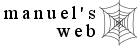nursing calculators nursing search site map

(Requires JavaScript on)

The following is a list of nursing calculators.  Each calculator, along with examples and formulas, provides a unique way to review nursing math.  These calculators are featured on Nurseweek.com and soon to be part of Nursingbar.com.

## Directions

Each link below, describing the calculator, will take you to that calculator's web page.  To work these calculators, everything above the "solve" button is for you to fill in.  The answer(s) will be shown below the "solve" button.  Friendly alerts will appear if you leave a box blank or type in something wrong.  You may see examples of the calculator on those pages (examples shown are just for display).

 This calculator does not work. It is just for display. These radio buttons let you choose between options. Click and point with your mouse to select or change an option. The dot indicates which option is selected.All the boxes above the "solve" button are for you to fill in. These boxes require numbers or decimal points (periods) only. The "solve" button takes whatever information is entered and calculates the result. All boxes below the "solve" button are where the results are displayed.  Don't put anything here. The "reset" button clears all information entered. The radio buttons will go back to their default option.

## Dosage calculators

1. PO dosage calculator - solids  i.e. tablets, caplets, capsules, lozenges
2. PO dosage calculator - liquids    calculates liquid medications such as acetaminophen elixir
3. IV dosage calculator    calculates IV medications such as furosemide and digoxin

## Calculate IV rates

1. IV rate (gtt/min)    calculates IV rates without using an IV pump
2. IV rate (mL/hr)    calculates IV rates when given a time limit when IV must be infused
3. mcg/min as mL/hr    calculates IV medication rates such as norepinephrine and nitroglycerine
4. mcg/kg/min as mL/hr    calculates IV medication rates such as nitroprusside and dopamine

## Calculate infusion time

1. IV time (hrs)     given an IV rate, calculates the duration of an IV

## Calculate dosage given or infusing

There are times when the only way to figure out how much medication is being infused is by looking at the information on the IV bag and the rate of the infusion.

1. mL/hr as dose/hr    calculates the dose of medications infusing such as heparin
2. mL/hr as mcg/min    calculates the dose of medications infusing such as nitroglycerine and norepinephrine
3. mL/hr as mcg/kg/min     calculates the dose of medications infusing such as dobutamine and nitroprusside

## Clinical Calculators

1. pediatric dose calculator    converts an adult dosage into a pediatric using BSA
2. O2 remaining in e-cylinder    calculates how much O2 is left according to rate infusing
3. bsa calculator     calculates the BSA according to height and weight
4. drug absorption (Pka)     determines how well a medication will be absorbed into the body
5. O.R. fluid requirements     calculates IV rate according to the type of surgery
6. N.P.O. fluid replacement    calculates fluid replacement for time NPO
7. allowable blood loss    calculates how much blood can be lost without needing a transfusion
8. estimated blood volume    estimates how much blood is in a person's body
9. Ideal Body Weight (IBW)    compares an individuals weight against a statistical norm
10. Adjusted Body Weight (ABW)    calculates the ABW in obese persons for more accurate drug dosing
11. ABG interpreter    interprets a respiratory or metabolic cause for pH disorders.

## Conversion Calculators

1. °F,°C conversions    converts Fahrenheit and Celsius temperatures
2. pounds to kilograms    converts pound and kilogram weights
3. inches to centimeters    converts inch and centimeter lengths
4. foot, inch to centimeters    converts foot, inch and centimeter lengths (i.e 5'3" = 160 cm)
5. non-metric to metric    converts gr, tsp, tbs, oz, cup, min, dr to metric
6. metric units   converts between metric weights and volumes
7. % solutions to mg/mL    converts % solution into mg/mL concentration
8. epinephrine solutions to mg/mL    chart that converts a ratio into a mg/mL concentration

## Drug  Calculators

1. Propofol bolus/infusion    calculates how many mL to push for intubation or rates for sedation and maintenance
2. Muscle relaxants    calculates how many mL to push for intubation

Survey

Survey    Rate my calculators choose from "very useful," "useful," "somewhat useful," or "not useful."